# The train

The train passed the observer in the 20s and passed through a 1500m tunnel in 2 minutes. Calculate the speed (km/h) and train length.

v =  54 km/h
l =  300 m

### Step-by-step explanation:

v• (20/3600) = s
1500/1000 + s = v•2/60

3600s-20v = 0
3000s-100v = -4500

s = 3/10 = 0.3
v = 54

Our linear equations calculator calculates it.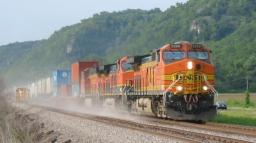Did you find an error or inaccuracy? Feel free to write us. Thank you!Tips to related online calculators
Do you have a linear equation or system of equations and looking for its solution? Or do you have a quadratic equation?
Do you want to convert length units?
Do you want to convert velocity (speed) units?
Do you want to convert time units like minutes to seconds?

## Related math problems and questions:

• Two trainsThe train runs at speed v1 = 72 km/h. The passenger, sitting in the train, observed that a train long l = 75m in 3 s passed on the other track in the opposite direction. Calculate the speed of this train.
• Train speedTwo guns were fired from the same place at an interval of 10 minutes and 30 seconds, but a person in a train approaching the place hears second shot 10 minutes after the first. The speed of the train (in km/hr), supposing that sound travels at 340 m/s is:
• Car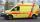The car drove some distance in 3 hours and 20 minutes. If it increases speed by 10 km/h, it goes this distance in 2.5 hours. Calculate the distance.
• Two trainsThrough the bridge, long l = 240m, the train passes through the constant speed at time t1 = 21s. A train running along the traffic lights at the edge of the bridge passes the same speed at t2 = 9s. a) What speed v did the train go? b) How long did it take
• TrainsFrom station 130 km away started passenger train and after 2.2 hours after the express train, which travels 37 km an hour more. Express train finish journey 7 minutes early. Calculate the average speed of this two trains.
• Train speed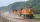The train speed is decreased during 50 sec from 72 km/h to 36 km/h. Assuming that the train movement is equally slowing, find the the acceleration and the distance that it travels at.
• Two trains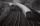There are two trains running the same distance. 1st train will travel it in 7 hours 21minutes. 2nd the train will travel 5 hours 57minutes and 34 seconds and it is 14 km/h faster than the first train. What are speeds of trains and how long is this railway
• Motion 2At 10 o'clock started from place A car speed at 90 km/h. 20 minutes after started from the place B at speed 60 km/h another car. Distance places A and B is 120 kilometers. What time and where they meet?
• Ascent and descent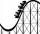Car goes 114 km track, which consists of ascent and descent at time of 1 hour 35 minutes. When climbing moves at the speed 48 km/h and downhill 25 m/s. What is the length of climb and descent?
• Average speed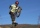The average speed of a good cyclist is 30 km/h. The average speed of the less able is 20 km/h. They both set off on the same route at the same time. Good cyclist drove it 2 hours earlier. How long was the route?
• Two cyclistsTwo cyclists started from crossing in the same time. One goes to the north speed 20 km/h, the second eastward at speed 26 km/h. What will be the direct distance cycling 30 minutes from the start?
• The cyclist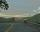The cyclist went from village to town. The first half of the journey went at 20 km/h. The second half of the journey, which mostly fell, went at 39 km/h. All journey took 88 minutes. Calculate the distance from the village to the town.
• Truck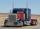Truck went for 4 hours and 30 minutes. If truck increase speed by 11 km/h, went same distance in 2 hours. Calculate this distance.
• Two friendsTwo friends on a bicycle rode against each other from two places 49 km apart. The first set off at 8.00 at a speed of 20km/h, the second 12 minutes later at a speed of 25km/h. What time do they meet? How many km will each of them travel since then?
• Cars speedsDistance from city A to city B is 108 km. Two cars were simultaneously started from both places. The speed of a car coming from city A was 2 km/h higher than the second car. What was the speed of each car if they met in 54 minutes?
• Two portsFrom port A on the river, the steamer started at an average speed of 12 km/h towards port B. Two hours later, another steamer departed from A at an average speed of 20 km/h. Both ships arrived in B at the same time. What is the distance between ports A an
• TruckIn 7 hours started from town Krnov truck at speed 40 km/h. Passenger car started against it in 8 hours 30 minutes from the city of Jihlava at speed 70 km/h. Distance between this two cities is 225 km. At what time and at what distance from Krnov this two